# Difference between revisions of "0708-1300/Class notes for Tuesday, February 26"

Announcements go here

## A Homology Theory is a Monster

Bredon's Plan of Attack: State all, apply all, prove all.

Our Route: Axiom by axiom - state, apply, prove. Thus everything we will do will be, or should be, labeled either "State" or "Prove" or "Apply".

## Typed Notes

The notes below are by the students and for the students. Hopefully they are useful, but they come with no guarantee of any kind.

### First Hour

Recall we had defined for a chain complex the associated homology groups: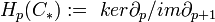$H_p(C_*) :=\ ker\partial_p/im\partial_{p+1}$

From this we get the pth homology for a topological space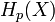$H_p(X)$

We have previously shown that

1)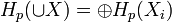$H_p(\cup X) = \oplus H_p(X_i)$ for disjoint unions of spaces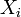$X_i$

2)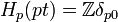$H_p(pt) = \mathbb{Z}\delta_{p0}$

3)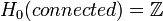$H_0(connected) = \mathbb{Z}$

4)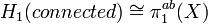$H_1(connected) \cong \pi_1^{ab}(X)$ via the map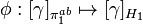$\phi:[\gamma]_{\pi_1^{ab}}\mapsto[\gamma]_{H_1}$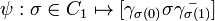$\psi:\sigma\in C_1\mapsto[\gamma_{\sigma(0)}\sigma\bar{\gamma_{\sigma(1)}}]$ where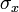$\sigma_x$ is a path connecting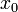$x_0$ to x.

We need to check the maps are in fact inverses of each other.

Lets consider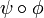$\psi\circ\phi$. We start with a closed path starting at$x_0$ (thought of as in the fundamental group).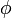$\phi$ means we now think of it as a simplex in X with a point at$x_0$.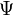$\Psi$ now takes this to the path that parks at$x_0$ for a third of the time, goes around the loop and then parks for the remaining third of the time. Clearly this is homotopic this composition is homotopic to the identity.

We now consider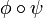$\phi\circ\psi$. Start with just a path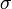$\sigma$. Then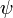$\psi$ makes a loop adding two paths from the$x_0$ to the start and finish of$\sigma$ forming a triangular like closed loop. We think of this loop as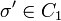$\sigma'\in C_1$

Now, we start from c being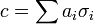$c = \sum a_i\sigma_i$ with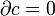$\partial c = 0$. So get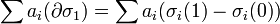$\sum a_i(\partial \sigma_1) = \sum a_i(\sigma_i(1)-\sigma_i(0))$

So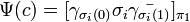$\Psi(c) = [\gamma_{\sigma_i(0)}\sigma_i\bar{\gamma_{\sigma_i(1)}}]_{\pi_1}$ which maps to, under$\phi$,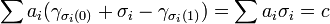$\sum a_i(\gamma_{\sigma_i(0)} + \sigma_i - \gamma_{\sigma_i(1)}) = \sum a_i\sigma_i = c$ ( in the homology gamma's cancel as$\partial c = 0$)

Axiomized Homology

We now will move to an approach where we prove that our defined homology satisfies a series of established homology axioms that will allow us to apply the machinery of general homology to our specific "singular" homology defined via simplexes.

Axiom 0) Homology if a function

Definition The "category of chain complexes" is a category whose objects are chain complexes (of abelian groups) and morphisms which is a homomorphism between each abelian group in one chain and the corresponding group in the other chain such that the resulting diagram commutes. I.e,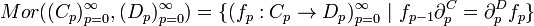$Mor((C_p)_{p=0}^{\infty}, (D_p)^{\infty}_{p=0}) = \{(f_p:C_p\rightarrow D_p)_{p=0}^{\infty}\ |\ f_{p-1}\partial_p^C=\partial_p^D f_p\}$

Now, in our case, the chain complexes do in fact commute because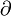$\partial$ is defined by pre-composition but f is defined by post-composition. Hence, associativity of composition yields commutativity.

Claim

Homology of chain complexes is a functor in the natural way. That is, if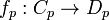$f_p:C_p\rightarrow D_p$ for each p induces the functor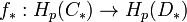$f_*:H_p(C_*)\rightarrow H_p(D_*)$

The proof is by "diagram chasing". Well, let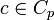$c\in C_p$,$\partial c =0$.

Let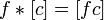$f*[c] = [fc]$. Now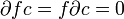$\partial fc = f\partial c = 0$. Furthermore, suppose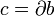$c = \partial b$. Then,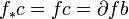$f_*c = fc = \partial fb$ so therefore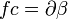$fc = \partial\beta$ some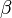$\beta$. This shows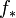$f_*$ is well defined.

Thus, for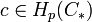$c\in H_p(C_*)$ get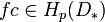$fc\in H_p(D_*)$ via the well defined functor$f_*$.

### Second Hour

1) Homotopy Axioms

If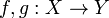$f,g:X\rightarrow Y$ are homotopic then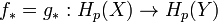$f_* = g_*: H_p(X)\rightarrow H_p(Y)$

Applications: If X and Y are homotopy equivalent then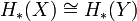$H_*(X) \cong H_*(Y)$

Proof:

let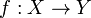$f:X\rightarrow Y$,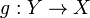$g:Y\rightarrow X$ such that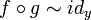$f\circ g\sim id_y$ and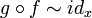$g\circ f \sim id_x$. Well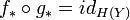$f_*\circ g_* = id_{H(Y)}$ and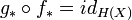$g_*\circ f_* = id_{H(X)}$

Hence,$f_*$ and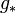$g_*$ are invertible maps of each other. Q.E.D

Definition

Two morphisms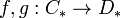$f,g:C_*\rightarrow D_*$ between chain complexes are homotopic if you can find maps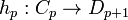$h_p:C_p\rightarrow D_{p+1}$ such that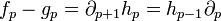$f_p - g_p = \partial_{p+1} h_p = h_{p-1}\partial_p$

Claim 1

Given H a homotopy connecting f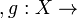$,g:X\rightarrow$ Y we can construct a chain homotopy between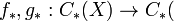$f_*,g_*:C_*(X)\rightarrow C_*($Y)

Claim 2

If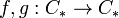$f,g:C_*\rightarrow C_*$ are chain homotopic then they induce equal maps on homology.

Proof of 2

Assume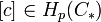$[c]\in H_p(C_*)$, that is,$\partial c =0$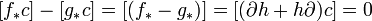$[f_* c] - [g_* c] = [(f_*-g_*)] =[(\partial h + h\partial)c] = 0$ (as$\partial c = 0$ and homology ignores exact forms)

Hence, at the level of homology they are the same.

Proof of 1

Consider a simplex in X. Now consider its image, a simplex, in Y under g and f respectively. Because of the homotopy we can construct a triangular based cylinder in Y with the image under f at the top and the image under y at the bottom.

Define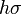$h\sigma$ = the above prism formed by$\sigma$ and the homotopy H.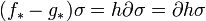$(f_*-g_*)\sigma = h\partial\sigma = \partial h\sigma$

This, pictorially is correct but we need to be able to break up the prism,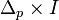$\Delta_p\times I$ into a union of images of simplexes.

Suppose p=0, i.e. a point. Hence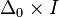$\Delta_0\times I$ is a line, which is a simplex.

Suppose p=1 which yields a square. Adding a diagonal divides the square into two triangles, so is clearly a union of simplexes.

Suppose p=2. We get a prism which has a triangle for a base and a top. Raise each vertex on the bottom to the top in turn. This makes the prism a union of three simplexes.

In general for$\Delta_p\times I$ let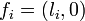$f_i = (l_i,0)$ and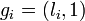$g_i = (l_i,1)$ for vertexes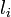$l_i$

Then,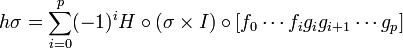$h\sigma = \sum_{i=0}^p (-1)^i H\circ(\sigma\times I)\circ[f_0\cdots f_i g_i g_{i+1}\cdots g_p]$

which is in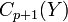$C_{p+1}(Y)$

So have maps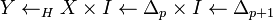$Y\leftarrow_H X\times I\leftarrow \Delta_p\times I \leftarrow\Delta_{p+1}$

Claim: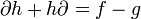$\partial h +h\partial = f-g$

Loosely,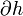$\partial h$ cuts each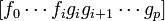$[f_0\cdots f_i g_i g_{i+1}\cdots g_p]$ between the f_i and g_i and then deletes an entry. h\partial however does these in reverse order. Hence all that we are left with is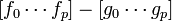$[f_0\cdots f_p] - [g_0\cdots g_p]$